Search
Search

# (Very) Basic Intro to Lattices for Post Quantum CryptographyBy Lane Wagner – @wagslane on Twitter

Lattice-based cryptography has been coming into the spotlight recently. In January 2019, Many of the semifinalists in the NIST post-quantum-cryptography competition were based on lattices. Let’s explore the basics of lattices and how they apply to cryptosystems.

## What is a Lattice?

According to Wikipedia, a lattice is the set of all integer linear combinations of basis vectors:

i.e.

More simply put, a lattice is defined by basis vectors, which are only able to be scaled by integers… yay no fractions!

For example, let’s create a lattice of all the integers in a two-dimensional plane:

The definition of our lattice contains only 2 basis vectors,

v1 = (0,1)

v2 = (1,0)

Our lattice is the set of all values that can be reached by any combination and scale of our basis vectors. For example, the point (2,0) is in our lattice because it can be reached by 2*v1

Similarly, we could create an entirely new lattice by changing our basis vectors to

v1 = (0,3)

v2 = (3,0)

As you can see, now the intermediary points (0,1) and (0,1) no longer exist in our lattice. There is no way to scale v1 (0,3) and v2 (3,0) to reach those points without using fractional scalars. With lattices, we can only scale by whole integers.

## How Does This Help With Crypto?

Cryptographic algorithms are typically based on mathematical problems that are easy to verify the answer of, but hard to calculate.

For example, RSA is based on prime factorization. If I told you to find prime factors of 27,919,645,564,169,759, that would be hard. However, if I told you that 48,554,491 and 575,016,749 are prime factors, all you have to do is multiply them together in order to verify my answer.

RSA works great with classical computers. There are no known solutions to find prime factors of a number reliably in less than exponential time.

## Shortest Vector Problem

In this introductory article, we will take a brief look at one of the more well-known lattice problems that are of use in cryptosystems, the shortest vector problem (SVP).

Simply put, the goal of SVP is for the attacker to find the shortest vector from the origin (above in red) when given the basis of a lattice (above in blue). A zero vector doesn’t work as an answer, we consider it trivial.

How is it solved?

Like RSA with classical computers, it is hard to find the shortest vector of a large lattice, especially if it exists in many dimensions. One such slow solution for approximating the shortest vector is Babai‘s algorithm, or Nearest Plane Algorithm, which you can read about in the links provided. This post originally appeared on Qvault

Lane on Dev.to: wagslane

Subscribe To Qvault: https://qvault.io

## Recommended WatchingThe Future of Materials Discovery: Reducing R&D Costs significantly with GenMat’s AI and Machine Learning Tools

When: July 13, 2023 at 11:30am

What: GenMat Webinar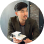#### Jake Vikoren

Company Speaker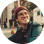Company Speaker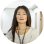#### Araceli Venegas

Company Speaker## Relevant

### Toshiba Digital Solutions and Classiq to Collaborate on Gate-based Quantum Computing## RecommendedThe Future of Materials Discovery: Reducing R&D Costs significantly with GenMat’s AI and Machine Learning Tools

When: July 13, 2023 at 11:30am

What: GenMat Webinar#### Jake Vikoren

Company SpeakerCompany Speaker#### Araceli Venegas

Company SpeakerKeep track of everything going on in the Quantum Technology Market.

In one place.

## Related Articles## July 28, 2021## January 5, 2021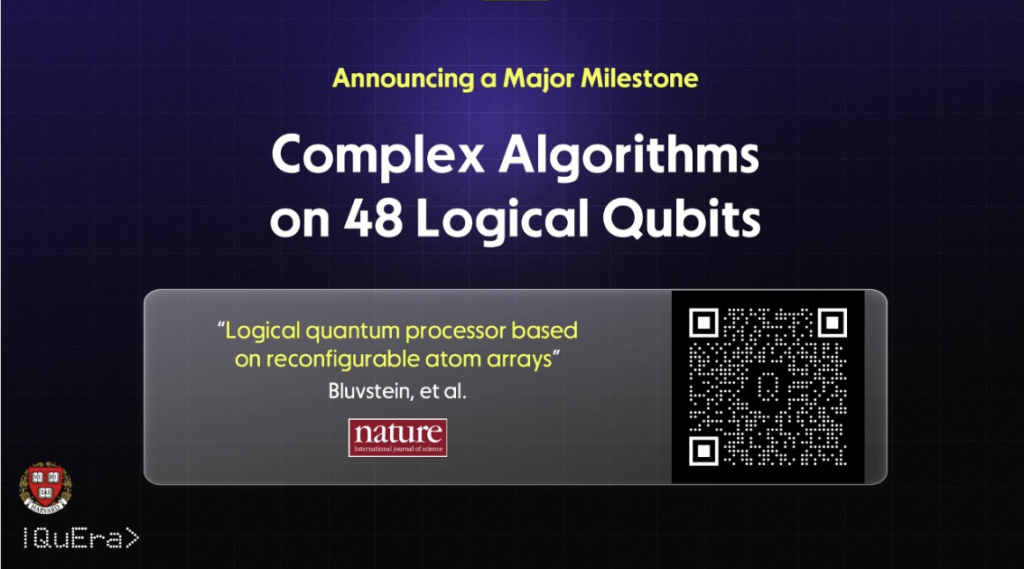## December 6, 2023## December 6, 2023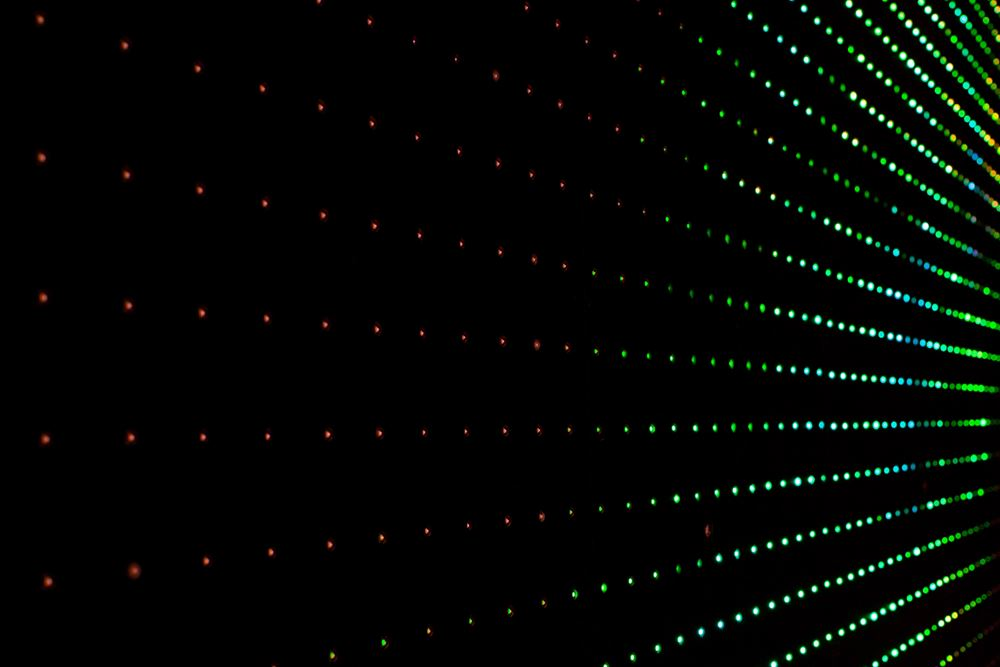## December 7, 2023## December 5, 2023

Explore our intelligence solutions